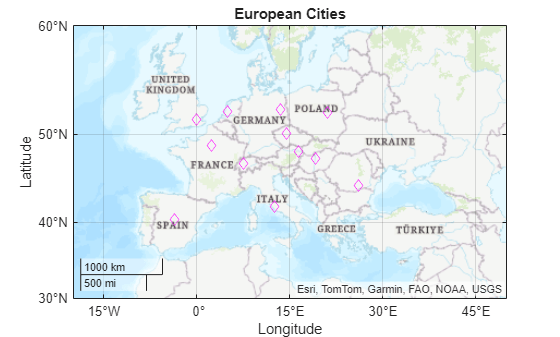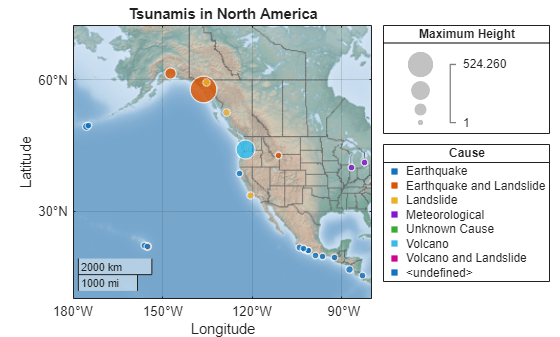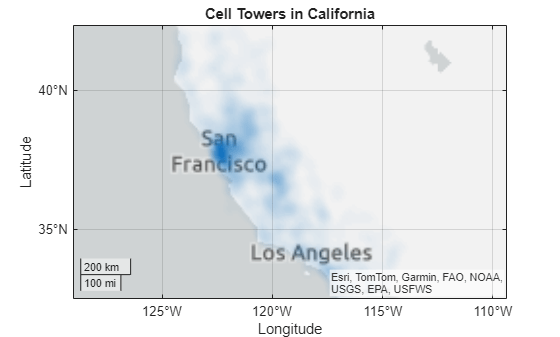# Plot in Geographic Coordinates

If you have data that is associated with specific geographic locations, use a geographic axes or chart to visualize your data on a map and provide visual context. For example, if you have data that describes the occurrences of tsunamis around the world, plot the data in a geographic axes where a marker indicates the location of each occurrence on a map. These examples show how to create line plots, scatter plots, bubble charts, and density plots in geographic coordinates.

### Create Geographic Line Plot

Draw a line on a map between Seattle and Anchorage. Specify the latitude and longitude for each city, then plot the data using the `geoplot` function. Customize the appearance of the line using the line specification `'g-*'`. Adjust the latitude and longitude limits of the map using `geolimits`.

```latSeattle = 47.62; lonSeattle = -122.33; latAnchorage = 61.20; lonAnchorage = -149.9; geoplot([latSeattle latAnchorage],[lonSeattle lonAnchorage],'g-*') geolimits([45 62],[-149 -123])```### Create Geographic Scatter Plot

Create latitude and longitude positions and define values at each point. Plot the values on a map using the `geoscatter` function. The example specifies the triangle as the marker, with size and color representing variations in the values.

```lon = (-170:10:170); lat = 50 * cosd(3*lon); A = 101 + 100*(sind(2*lon)); C = cosd(4*lon); geoscatter(lat,lon,A,C,'^')```### Create Geographic Bubble Chart

Create a table from tsunami data. Define one value as a categorical value. Plot the data on a map using the `geobubble` function. The example uses the size of the bubble to indicate the height of the tsunami wave and color to indicate the cause of the tsunami.

```tsunamis = readtable('tsunamis.xlsx'); tsunamis.Cause = categorical(tsunamis.Cause); figure gb = geobubble(tsunamis,'Latitude','Longitude', ... 'SizeVariable','MaxHeight','ColorVariable','Cause'); geolimits([10 65],[-180 -80]) title 'Tsunamis in North America'; gb.SizeLegendTitle = 'Maximum Height';```### Create Geographic Density Plot

Create a table from tsunami data. Plot the data using the `geodensityplot` function.

```tsunamis = readtable('tsunamis.xlsx'); lat = tsunamis.Latitude; lon = tsunamis.Longitude; weights = tsunamis.MaxHeight; geodensityplot(lat,lon,weights) geolimits([10 65],[-180 -80])```